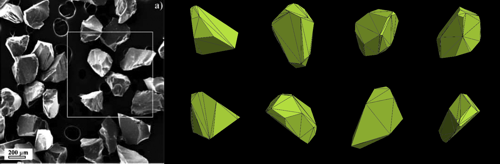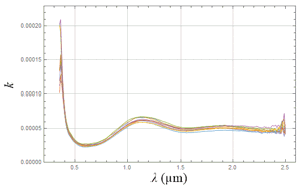Europlanet Science Congress 2020
Virtual meeting
21 September – 9 October 2020
EPSC Abstracts
Vol. 14, EPSC2020-381, 2020, updated on 25 May 2021
https://doi.org/10.5194/epsc2020-381
Europlanet Science Congress 2020

# Rigorous inversion of absorption coefficient from spectral properties of particulate material

Antti Penttilä et al.

The optical constant of the material, meaning the complex refractive index m=n+i k, is an essential parameter when considering the reflection and absorption properties of that material. The refractive index is a function of wavelength of the light, and usually the imaginary part k is what governs the reflection or transmission spectral behavior of the material.

The knowledge of the complex refractive index as a function of wavelength, m(λ), is needed for light scattering simulations. On the other hand, rigorous scattering simulations can be used to invert the refractive index from measured or observed reflection spectra. We will show how the combination of geometric optics and radiative transfer codes can be used in this task.

In this work, the possible application is with the future visual-near infrared observations of Mercury by the ESA BepiColombo mission. That application in mind, we have used four particulate igneous glassy materials with varying overall albedo and in several size fractions in reflectance spectra measurements (hawaiitic basalt, two gabbronorites, anorthosite, see details from Carli et al, Icarus 266, 2016). The grounded material consist of particle with clear edges and quite flat facets, and we choose to model the particle shapes by geometries resulting from Voronoi division of random seed points in 3D space (see Fig. 1).Figure 1. On the left, a SEM image of anorthosite particles, size fraction 224–250 µm (from Carli et al, Icarus 266, 2016). On the right, eight example particles from Voronoi division algorithm.

The refractive index inversion is done here using first a geometric optics code SIRIS (Muinonen et al, JQSRT 110, 2009) to simulate the average Mueller matrix, albedo, and scattering efficiency for a single Voronoi particle. Then, these properties are fed into radiative transfer code RT-CB (Muinonen, Waves in Random Media 14, 2004) to produce the reflective properties of a semi-infinite slab of these particles. This procedure is repeated for a 2D grid of particle size parameters x=2πr/λ, where r is the radius of particle, and imaginary part k of refractive index. In Vis-NIR wavelengths, the real part n is quite constant and is estimated to be about 1.58 for all the four glasses. From the simulated slab reflectance data with the 2D x, k parameter grid, we can first interpolate, and then invert the k parameter for any reflectance value with given wavelength and particle size.

The resulting spectral behavior of k for the four glasses and for all the size fractions was seems very realistic. Carli et al. (Icarus 266, 2016) inverted the k spectral behavior for these same samples using Hapke modeling, and the results are quite similar. An example of inverted k for one sample (anorthosite) is shown in Fig. 2.Figure 2. Inverted imaginary part of refractive index, k, as a function of wavelength for different size fractions of anorthosite.

We show that rigorous light scattering simulations can be used to invert the optical constant of a material from observed reflectance spectra, at least if some assumptions regarding particle sizes can be done. These methods can be valuable when analyzing various illumination and observing geometries, intimate mixtures of multiple materials, and other cases where Hapke modeling might not be enough for accurate inversion. We foresee employing these methods to analyze the observations of Mercury from ESA BepiColombo mission.

Acknowledgements: Research supported, in part, by the Academy of Finland (project 325805).

How to cite: Penttilä, A., Väisänen, T., Martikainen, J., Carli, C., Capaccioni, F., and Muinonen, K.: Rigorous inversion of absorption coefficient from spectral properties of particulate material, Europlanet Science Congress 2020, online, 21 Sep–9 Oct 2020, EPSC2020-381, https://doi.org/10.5194/epsc2020-381, 2020.